# Lab 4: Sensors!

## Topics

1. First Build
2. Hardware Theory
3. Software Theory
4.  Exercises
5. References

## 1. First Build

Take a few moments to build this circuit and install the sketch code. You will know you got things right if you hear weird noise coming from the piezo that changes and sounds "video-gamey" as you twist the potentiometer. The LED will be off most of the time but may flicker occasionally. If you have an Arduino Mega the tone will be pure and the LED will change brightness as you turn the potentiometer.

### 1.1 The Circuit

This circuit isn't too complicated. Pin A0 should be connected to the middle pin of the potentiometer. For everything else come up with your own interpretation of the schematic below.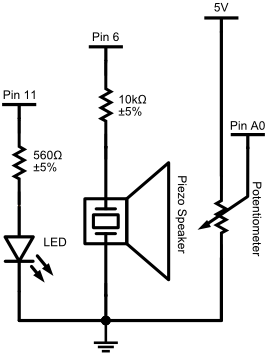### 1.2 The Code

``````
/*
Playing with pots.
Written 23 Aug 2011 by Alex Clarke
*/

//constants for this sketch
const int ledPin = 11;
const int piezoPin = 6;
const int potPin = A0;

// variables for this sketch
int pot_value;
int frequency;

void setup()
{
pinMode(ledPin, OUTPUT);
pinMode(piezoPin, OUTPUT);
}

void loop()
{

//Set the LED to brightness pot_value
analogWrite(ledPin, pot_value);

//Play the sound represented by frequency pot_value
tone(piezoPin, pot_value);
}
``````

## 2. Hardware Theory

The Arduino's six analog inputs are able to sense different voltage levels and convert them to numbers you can use in your sketch. Some input devices provide voltages you can use directly. Others require creative use of Ohm's law and the concept of voltage division to use with your Arduino. Some devices provide analog values based on time rather than voltage and require additions to the Arduino language to use. This lab we will use voltage division and a couple related devices. Next lab you will explore some of the other types.

The lab demo will show how to use a multimeter like this one to help measure the properties of your circuits: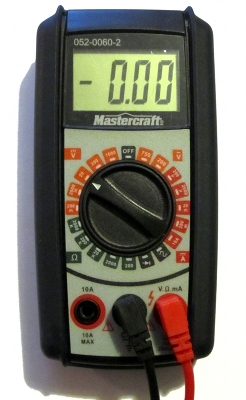### 2.1 Ohm's Law

You should recall from class that Ohm's law relates Voltage, Current and Resistance like this:

Let:

V be voltage in Volts
I   be current in Amps
R be resistance in Ohms.

Then:

V = I R

You should also have been shown the following diagram that helps you know how to rearrange Ohm's Law to solve for different parts of the equation:You will be directly applying this law in this lab.

### 2.2 Voltage Division

Voltage division is an effect seen in simple series circuits with multiple resistive components.  Between each component of the circuit you can measure a different voltage that is related to the total resistances before and after that point in the circuit.

A series circuit in a stable state has one current value that is constant from end to end. The value of this current can be determined with Ohm's law, using the total resistance of all components in the circuit.

Over the circuit the voltage will drop from high to low in the direction of the current. On your Arduino this means it will drop from +5V to 0V. The voltage drops as it passes through resistive components in the circuit. The amount the voltage drops across each of these components can be calculated using Ohm's law. We then can calculate a voltage value for the wires between components in the circuit.

Let's combine these two concepts to calculate the voltages between the resistors in the following circuit. You may assemble the circuit and verify the calculations with your multimeter if you wish.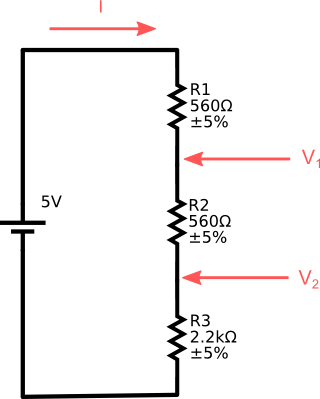First, calculate the current for this circuit:

I = V / R
= 5V / (560Ω + 560Ω + 2200Ω)
= 5V / 3320Ω
≈ 0.001506A
≈ 1.506mA

You can confirm this with your multimeter:

• Set it to either 2000µ or 20m.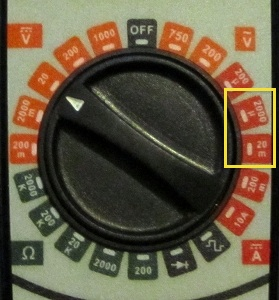• The first represents currents up to 2mA or 2000µA.
• The second represents currents up to 20mA.
• Current is measured in a circuit by inserting the leads of the multimeter into the circuit.
• Disconnect the ground wire from your circuit.
• Touch the red probe to the end of the last resistor in your circuit.
• Touch the black probe to the grounding wire.
Second, calculate the voltage drop for each resistor:

VR1 = I R1
≈ 0.001506A × 560Ω
≈ 0.8434V

VR2 is the same as VR1

VR3 = I R3
≈ 0.001506A × 2200Ω
≈ 3.313V

You can confirm each of these voltage drops with your multimeter:
• Set it to either 20 in the DC Voltage section (V with Solid and dashed lines above).
• This represents voltages up to 20V.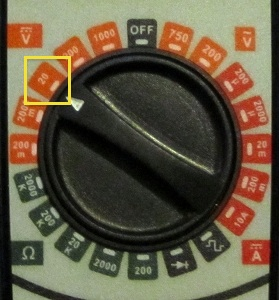• Touch the red and black probes to the positive and negative sides to wires in your active circuit on either side of where you want to measure a voltage difference or drop.

Last, we can calculate the expected remaining voltage at each point in the circuit. You can do this two ways:
1. Add together all the voltage drops that ahead of the measured point.
2. Subtract all the voltage drops before the measured point from the input voltage.
So if should get the input voltage, 5V, if we measure before any resistive components. Using method 1:

Vin ≈ 0.8434V + 0.8434V + 3.313V ≈ 5V

At point V1:

V1 ≈ 0.8434V + 3.313V ≈ 4.156V

At point V2:

V2 ≈ 3.313V

### 2.1 Potentiometers

Potentiometers are the component behind most knobs that you see on electronic devices. They work by the principle of voltage division to adjust output voltage between two reference levels.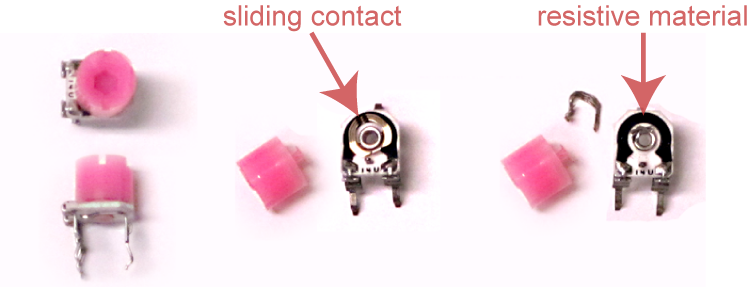Inside they have a length of resistive material, often graphite or a carbon/plastic mixture, that is connected to terminal wires at either end.  A sliding contact connected to a third terminal is moved back and forth across the resistive material, usually with some kind of knob or slider. The the resistance between the contact and the two end terminals is related to the distance to the terminals — longer is more resistive and shorter is less.

The resistive material in most potentiometers stays the same along its length. Its resistance is directly, or linearly, related to length. In other potentiometers, the resistive material is tapered or changes properties across its length. This type is used in audio applications and is usually more expensive. The amount of resistance in this type is usually logarithmic - it increases with a curve that matches human sound perception.

Some potentiometers are meant to be used often. They are rugged and can be fitted with large knobs. Others are small and are meant to be hidden away. These are called trimpots, and are used by experts to tune a complex circuit. They are not meant to be used often and will wear out if twisted frequently.

The small blue potentiometer in your ARDX kit is a linear trimpot.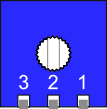The pins labeled 1 and 3 are should be connected to two different voltage levels. Pin 2, the middle pin, will have a voltage between the levels on pins 1 and 3. Turning the knob counterclockwise will adjust pin 2's voltage toward that of pin 1, and clockwise adjusts it toward that of pin 3. If that seems confusing, then try rotating your trimpot so that the pins are away from you. Then it should make more sense.

If you use your potentiometer to measure the Ohms between pins 1 and 3 the value should remain constant. If you measure from pin 1 or pin 3 to pin 2 the value will change as you rotate the knob. In an ideal potentiometer the total of the two should add up to the number from pin 1 to pin 3. In the real world, the total is always a little smaller because the contact is a conductor with a measurable width.

To use a potentiometer with your Arduino you usually connect:

• pin 1 to ground
• pin 3 to +5V

### 2.2 Photoresistors

Photoresistors are resistors that vary their resistance when exposed to light. They are less resistive under bright light than under dim light or in darkness. To use a photoresistor with your Arduino you must put it in series with a resistor to perform voltage division. A typical photoresistor sensor circuit would usually look like this: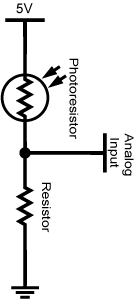The range of values you read at the analog input depend on the resistance properties of the photoresistor and the voltage division resistor you select. Once you know these, you should be able to calculate the range using Ohm's law.

### 2.3 Temperature Sensor

There are two three pin components in your ARDX kit. One is a transistor. The other, pictured below, says TMP 36GZ on the flat side of its case and is actually a complicated integrated circuit (IC) that can sense temperature. If you put it in a 5V circuit it will provide a voltage based reading that is related to its temperature.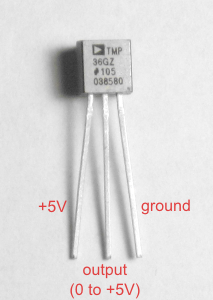You need to know some properties of the sensor (datasheet here) to convert its output to a temperature in degrees:

• Conversion factor: A 10mV change in this component is equivalent to a 1°C change in temperature. That means there's 0.01 V/°C. To convert from Volts to Celsius you need to invert that value: 100°C/V
• Offset: 0V represents -50°C. We have an offset of -50°C.
This suggests the following equation:
temperature_in_celsius = output_in_volts * conversion_factor  - offset
temperature_in_celsius = output_in_volts * 100 - 50

### 2.4 Touch Sensor (Capacitive Sensing)

It is possible to use a pair of digital inputs on your Arduino to detect if someone is near to or touching a metal plate. If you use the right combination of resistors, metal and insulators you can make a pressure sensor. This page on capacitive sensing at arduino.cc explains the theory behind the technique and gives a tutorial on how to build and use such a sensor. You will not have to build one in lab. Instead your lab instructor will provide one on an Arduino shield called the Danger Shield.

The ability to read a touch sensor is not built into the Arduino or its IDE. Instead you need to install and include a library - the Capacitive Sense library - to make it work. Please refer to the section on installing libraries to learn how to install the Capacitive Sense library.

## 3. Software Theory

### 3.1 The Tone Function

You can easily play notes on the piezo speaker in your kit with the tone() command. In its simplest form, a call to tone looks like this:
``````
tone(pin, frequency);
``````

The tone() command causes a note to begin to play on a pin at the specified frequency. The note will keep on playing while your program runs until another tone command is sent with a different frequency, or a noTone() command is sent.

There is a second form of tone() command that will stop automatically:

``````
tone(pin, frequency, milliseconds);
``````

As with the simple tone() command, your program will keep running while the note plays. If you want to wait for the sound to end before continuing with the program, you must use a delay() command of the same length as the tone. If you want silence between consecutive tones, you will need to use a longer delay.

Note: The tone() command has a nasty gotcha! It uses the PWM timers for pins 3 and 11 to make the note play automatically. Trying to use analogWrite() on those pins while you use the tone() command will cause strange things to happen. The first build sketch uses pin 11 to send analog writes to an LED which is causing interference. Change the sketch so it uses a different PWM pin. Enjoy the purer tones.

### 3.2 Printing to the Serial Monitor

Sometimes it's nice to be able to see what's going on inside your sketch. You can print values from your Arduino program to the computer and view them with the serial monitor in the Arduino software.

To begin sending messages to your computer you use the Serial.begin() command. You should use it in the setup() procedure. Try adding this to your setup():

``````
Serial.begin(9600); // 9600 baud is the default serial communication speed
``````

To send a message to the computer you use the Serial.print() or Serial.println() commands. They can print one variable or constant. They can also print a text message. Try adding this to your loop() just after the analogRead() command:

``````
Serial.print("I just read: "); //Print some text
Serial.println(pot_value);     //Print pot_value and go to next line
//delay(500);  //You may want to slow down the messages so you can keep up
``````

To see the resulting messages press the Serial Monitor button —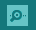— in your Arduino software. A window will pop up, your sketch will restart and text should appear. There's a menu in the lower right corner with a list of alternate speeds you can use with the Serial.begin() command.

Unlike with digital input, no pinMode() function call is needed to use analog input. All you need to do is call the analogRead(analogPin) function where analogPin is one of A0 through A5.

The analogRead() function returns an integer value between 0 and 1023 where 0 represents 0V and 1023 represents +5V. This is nice, but not helpful. You need to do some work to tell your Arduino how you want to respond to these values. Here are two basic strategies you can use to interpret analog input.

#### 3.3.1 Range Mapping

The map() function changes input values from one integer range to another. Here's a sample function call:

``````  result = map( input, fromLow, fromHigh, toLow, toHigh);

``````
• If input is equal to fromLow, result will be equal to toLow.
• If input is equal to fromHigh, result will be equal to toHigh.
• If input is somewhere in between fromLow and from High, it will be mapped to a corresponding place between toLow and toHigh.

For example, if you use raw values from the analog port with the tone() function, the results cover several octaves and includes frequencies lower than you can hear. The tone() function can't even reproduce the lowest tones properly. That's why this sketch sounds weird in some positions even after fixing the PWM pin conflict. The result is hard to control. You might have more control if you map analog values to one octave. The official reference note for modern music is A440 or 440Hz. One octave higher than that is A880 or 880Hz. We can map from (0,1023) to (440,880) to get exactly one octave.  Change your tone playing code from this:

``````
//Play the sound represented by frequency pot_value
tone(piezoPin, pot_value);

``````

to this:

``````
//Map pot_value to a usable frequency
int freq = map(pot_value, 0, 1023, 440, 880);
tone(piezoPin, freq);

``````

Note: the results of the map() function are integers or whole numbers. If you try to use map() to convert analog readings to volts, like this:

``float volts = map(pot_value, 0, 1023, 0, 5);``

then you will only get the numbers 0, 1, 2, 3 ,4 or 5. If you want more precise results you need to use the float type which allows decimal places. You could then calculate voltage like this:

``````
float volts = 5.0 * pot_value/1023.0;

``````

#### 3.3.2 Thresholding

A threshold is a value that splits a range of values into two parts — everything below and everything else or vice-versa. The following is an example of a threshold that you can try in your First Build sketch. Replace this code in your loop() procedure:
``````  //Set the LED to brightness pot_value
analogWrite(ledPin, pot_value);
``````

with this code:

``````  //Set a LED on/off threshold where the potentiometer passes the mid-point
if (pot_value < 512)
{
digitalWrite(ledPin, LOW);
}
else
{
digitalWrite(ledPin, HIGH);
}
``````

What this does is check to see if the voltage is less than halfway between 0V and +5V. That is 1023÷2 or 511.5.  When the potentiometer is exactly in the middle it should be near this value. If it is past it, the LED will turn on. If it is below it the LED will turn off.

You can also use a pair of thresholds to check if an input value lies inside a certain range. Change the above code as follows to light the LED when you are close to the midpoint on the pot:

``````  //Turn the LED on only if it is near the the mid-point
if (pot_value < 500 || pot_value > 524)
{
digitalWrite(ledPin, LOW);
}
else
{
analogWrite(ledPin, HIGH);
}
``````

It is better to use a range check like this than to check to see if your potentiometer is at an exact value using the == operator because it is very difficult to accurately position a potentiometer.

## 4. Exercises

### 4.1 Voltage Division (5 marks: 2 for demo, 3 for calculations and readings)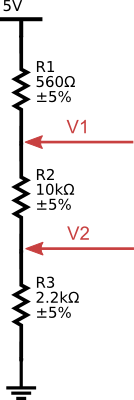• Remove the potentiometer and piezo speaker from your circuit and replace them with the above circuit.
• Write a voltmeter sketch:
• read analog values from A1
• convert them to float type volt values.
Hint: the formula is somewhere in the lab notes...
• print the result to the serial monitor
• use a long wire attached to A1 to probe the circuit at V1 and V2. Record your answers.
• In the lab:
• demonstrate your voltmeter and its circuit readings in volts to the lab instructor.
• demonstrate matching multimeter readings to the lab instructor
• write both sets of numbers down on paper or as a comment in your code
• After the lab:
• Submit your in-lab observations and confirming calculations for V1 and V2 to the Lab 4 assignment in URCourses.

### 4.2 Night Light (5 marks: 2 for demo, 3 for submitted code + documentation)

Now that you've mastered voltage division, use it to make your photo resistor control a night light.

• Create a circuit and sketch that turns on an LED when a shadow passes over a photoresistor. (See general circuit diagram in notes).
• It is up to you to choose what resistor to use with the photoresistor. Document your selection and circuit design. Use a diagram if necessary.
• It is up to you to select a threshold for turning on the LED
Hint: use the serial monitor...
• In the lab:
• demonstrate your night light and raw readings to the lab instructor.
• get a Danger Shield and get the nightlight sketch working on it
• After the lab:
• Tidy up your code - especially be sure the variable and constant names you choose are relevant.
• Document your nightlight circuit design, in the header or with a diagram.

#### 4.2a Danger Shield Nightlight

When you have demonstrated the Night Light sketch, your lab instructor will give you a Danger Shield. It is made and sold by SparkFun electronics. You will be using it a various times this semester because it includes ready to use sensors and outputs.

The Danger Shield has many of the same components found in your kit pre-wired to certain pins. Included are a couple simple LEDs and a photo resistor based sensor which is set up a lot like the one you used for your night light. Examine the photo below to see which pins they are on and adapt your Night Light sketch to the Danger Shield.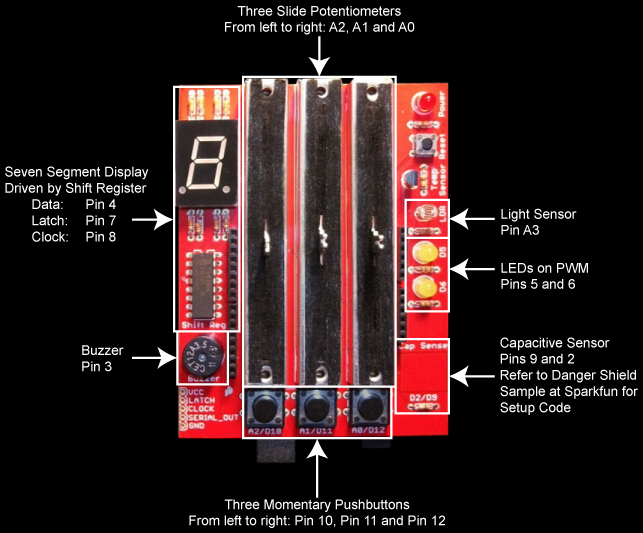Note: the Danger Shield may use a different resistor than you, and it may be set up backward to how you set it up. You might want to recalibrate your threshold.

### 4.4 Challenges (Bonus)

#### 12 Tone Arduino Instrument

Adapt the First Build sketch so that, as you turn the potentiometer, the piezo speaker only plays notes in the 12 tone (chromatic) equal tempered scale. Because this scale can be easily calculated you may use math to make the code compact, or you may use arrays to make it fast.

The frequencies for an equal tempered chromatic scale from C4 to C5 are:

 Note Freq. C4 C♯4/D♭4 D4 D♯4/E♭4 E4 F4 F♯4/G♭4 G4 G♯4/A♭4 A4 A♯4/B♭4 B4 C5 261.63 277.18 293.67 311.13 329.63 349.23 369.99 391.00 415.30 440.00 466.16 493.88 523.25

Beginner
Use your if/else skills to select the correct note to play.

Intermediate
Put the frequency values in an array, then use the `map()` function to calculate what index to use to look up frequencies in the array.

Use `map()` or some other calculation to determine the note number in a scale. Use this value as `n` in the the formula discussed in this Wikipedia article on Piano key frequencies.

### Deliverables

During the lab:
• Demonstration of completed sketches described in Parts 1 and 2.
By online submission before next lab:
• Calculations
• The code for all your sketches
• Document your night light circuit in the header for Part 2, or in a diagram if necessary.
(Optional) During this lab, in my office hours, or at the beginning of next lab:
• Your working solution to the 12 Tone Instrument challenge.